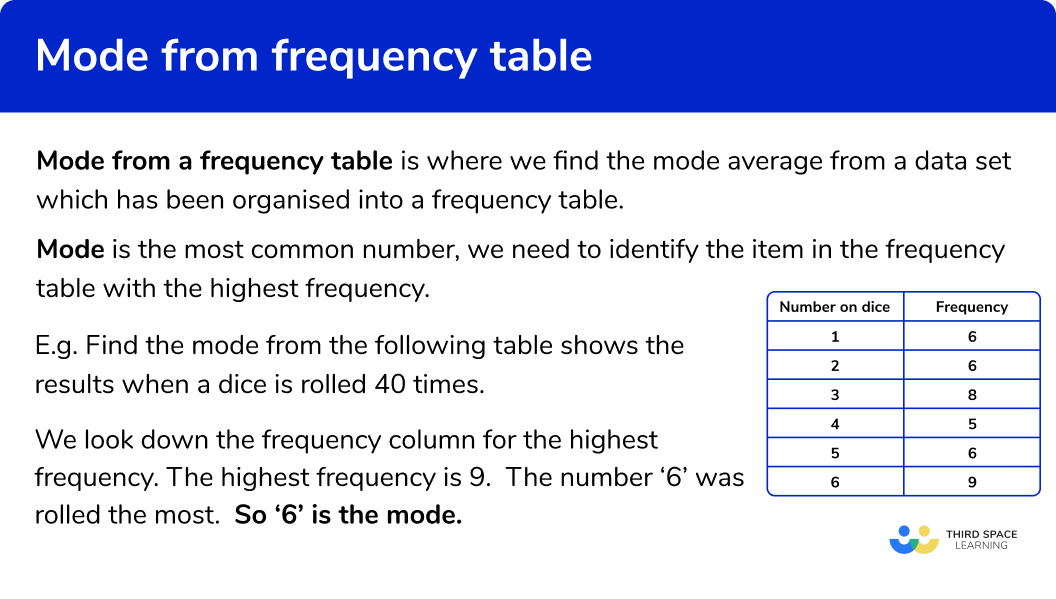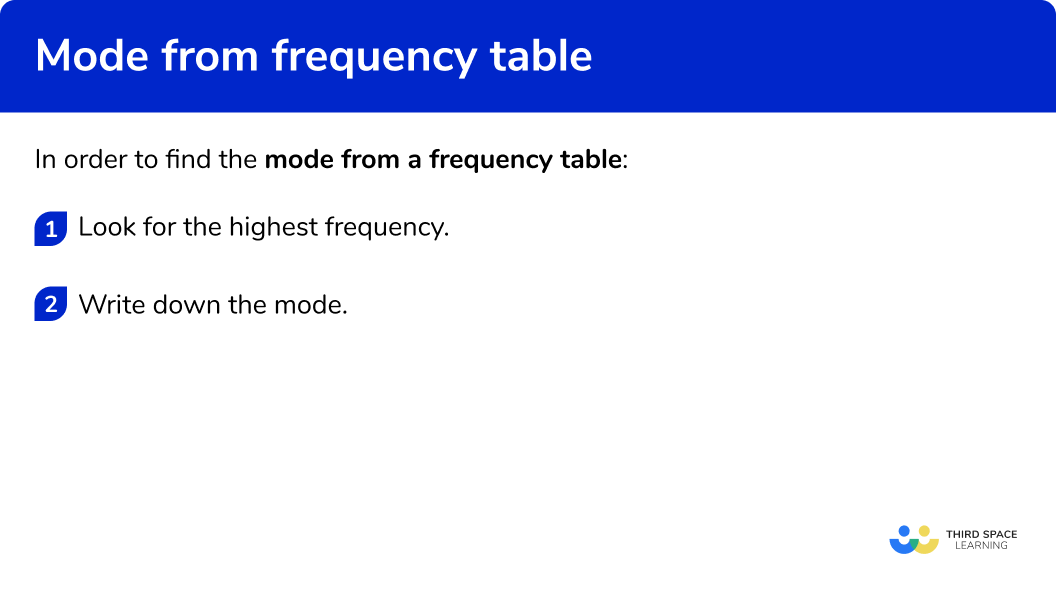GCSE Maths Statistics Frequency Tables

Mode from a Frequency Table

# Mode From A Frequency Table

Here we will learn about mode from a frequency table, including what the mode is and how to find the mode from a frequency table.

There are also averages from frequency tables worksheets based on Edexcel, AQA and OCR exam questions, along with further guidance on where to go next if you’re still stuck.

## What is mode from a frequency table?

Mode from a frequency table is where we find the mode average from a data set which has been organised into a frequency table.

E.g.

Mode is the most common number, in order to find the mode we need to identify the item in the frequency table with the highest frequency.

Mode is a measure of central tendency, it is a value that can be used to represent a set of data.

E.g.

Find the mode from the following table shows the results when a dice is rolled 40 times.

We look down the frequency column for the highest frequency. The highest frequency is 9, this means that the number ‘6’ was rolled the most.

So ‘6’ is the mode.

When the data is grouped and put into a grouped frequency table, the mode is called the modal class.

Step-by-step guide: Modal class (coming soon)

### What is mode from a frequency table?## How to find the mode from a frequency table

In order to In order to find the mode from a frequency table:

1. Look for the highest frequency.
2. Write down the mode.

### How to find the mode from a frequency table## Related lessons on frequency tables

Mode from a frequency table is part of our series of lessons to support revision on frequency tables. You may find it helpful to start with the main frequency table lesson for a summary of what to expect, or use the step by step guides below for further detail on individual topics. Other lessons in this series include:

## Mode from a frequency table examples

### Example 1: categorical data (words rather than numbers)

The frequency table shows the makes of 25 cars on a high street.

Find the mode from the following table:

1. Look for the highest frequency.

The highest frequency is 9

2Write down the mode.

The associated item with the highest frequency is ‘Ford’.

The mode is ‘Ford’

### Example 2: categorical data (words rather than numbers)

The frequency table shows the favourite fruit of a survey in a class of 28 students.

Find the mode from the following table:

The highest frequency is 11

The associated item with the highest frequency is ‘Banana’.

The mode is ‘Banana’

### Example 3: frequency table

The frequency table shows the number of goals scored by a football team in 44 matches over a year.

Find the mode from the following table:

The highest frequency is 15

The associated item with the highest frequency is ‘2’ .

The mode is ‘2’ goals

### Example 4: frequency table

The frequency table shows the shoe sizes in of 31 pairs in stock at a shop.

Find the mode from the following table:

The highest frequency is 8

The associated item with the highest frequency is ' 5\frac{1}{2} ' .

The mode shoe size is ' 5\frac{1}{2} '

### Example 5: grouped frequency table

The grouped frequency table shows the number of students in different 53 classes at a school.

Find the modal class from the following table:

The highest frequency is 15

The mode for a grouped frequency table is called the modal class. The associated class with the highest frequency is ‘29 32’.

The modal class is ‘29 32’.

### Example 6: grouped frequency table

The grouped frequency table shows the heights of 41 shrubs for sale in a garden centre.

Find the modal class from the following table:

The highest frequency is 13

The mode for a grouped frequency table is called the modal class. The associated class with the highest frequency is ‘50<x\leq 100’ .

The modal class is ‘50<x\leq 100’

### Common misconceptions

• Check which average you are being asked for

Check if you have been asked for the median, mode or mean average.

• Multiple modes?

A data set can have 1 mode or 2 modes (bimodal). It is possible that a data set has no mode at all.

• Item from the data set

The mode will be the item – not the frequency.

• Class intervals notation

The class intervals used in grouped frequency tables can be written in different ways. Take care when writing them, or finding the midpoints.

E.g.

0 to 5

0-5

0\leq x< 5

• Highest frequency

A value may appear more than once in a frequency column. This does not affect the mode. The mode is always indicated by the largest frequency, not the frequency that occurs the most often.

E.g.

The mode is 3 as this value has the highest frequency. (We do not need to consider the fact that the frequency ‘5’ appears twice in the frequency column).

### Practice mode from a frequency table questions

1. The frequency table shows favorite types of food as chosen by 32 students in a maths class.

Find the mode:Pizza138CurryThe highest frequency is 13. The associated item is ‘Curry’. So the mode is ‘Curry’.

2. The frequency table shows sizes of 27 t-shirts in stock at a shop.

Find the mode:SM98The highest frequency is 9. The associated item is ‘S’. So the mode is ‘S’.

3. The frequency table shows weights in grams of 70 packets of butter at a farm shop.

Find the mode:1825325113The highest frequency is 18 . The associated item is ‘251’. So the mode is ‘251’.

4. The frequency table shows the ages of 83 children in a rugby club.

Find the mode:2312189The highest frequency is 23. The associated item is ‘9’. So the mode is ‘9’.

5. The grouped frequency table shows the number of kittens in 34 litters.

Find the mode:144-67-912The highest frequency is 14. The associated item is ‘4-6’. So the modal class is ‘4-6’.

6. The grouped frequency table shows the number of lates from a class of 32 students.

Find the mode:170 to 420 to 2510The highest frequency is 17. The associated item is ‘0 to 4’. So the modal class is ‘0 to 4’.

### Mode from a frequency table GCSE questions

1. The table shows the type of 35 animals in a rescue centre.Write down the mode.

(1 mark)

Cat

(1)

2. The table shows the number of people living in 40 flats.Write down the modal number of people

(1 mark)

2

(1)

3. The table shows the heights of 70 students at a school.State the modal class interval.

(1 mark)

160\leq h<170

(1)

## Learning checklist

You have now learned how to:

• Find the mode from a frequency table
• Find the modal class interval from a grouped frequency table

## Still stuck?

Prepare your KS4 students for maths GCSEs success with Third Space Learning. Weekly online one to one GCSE maths revision lessons delivered by expert maths tutors.

Find out more about our GCSE maths tuition programme.